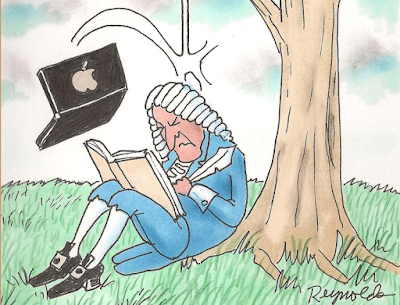### Would Newton Have Used Machine Learning?Sources: https://isaacnewtonassignmentufcc.weebly.com/newton-and-the-apple.html

As I was reading my Deep Learning book for my university course the other day, going through all the equations of how a linear regression machine learning algorithm reaches the parameters of a mathematical equation at 7:30am before work, with a good cup of Greek/Turkish coffee, a lightbulb went on in my head.

Let's rewind a bit back, for the past 2 weeks, I was already working on a machine learning algorithm to model the operation of an Air Handling Unit at work, and I was looking at complex mathematical/engineering equations which were supposed to be used to try and model the AHU operation.

So, that's when it clicked; why use very complex models with endless parameters, where real life is even much more complex to be modeled with equations from scratch, when in fact real-time data carries with it the complexity of the operations which, with a simple linear regression model and a few parameters, we can reach a good mathematical estimator to predict the operation of the AHU based on relatively little inputs (a couple of sensors and some weather data), just like how Edward Thorp did with blackjack?

So I set out to do just that, I opened my laptop, went to Jupyter Notebook and within a few hours built a replica of the AHU operations using machine learning (thanks to my colleague Elie who validated and fine-tuned it later on.) We then started feeding that model new artificial data to see how it would respond if we turned certain zones off, and the response was amazing, it was like we had a simulation software that can help us simulate operations off-line to find optimal operations and increase the building savings and estimate these savings, this really helped us make better decisions about the optimal operations of the machine.

And this got me asking, if Newton was living in our time, which machine learning algorithms would he have used to reach his formulas?

Cheers,

Edmond